# Colleges with the lowest SAT scores in Michigan

Top 10 colleges in Michigan with the lowest SAT scores
Looking for the colleges with the lowest SAT scores in Michigan? Well you're in luck! We've compiled a national college database and have created a list of the top 10 universities with the lowest SAT scores in Michigan below. If you are not a good test taker or worried about your test scores, this list is for you. These are the schools whose applicants had the lowest average SAT scores in Michigan, which means that you can get into these colleges with a lower SAT score. We also include each college's ACT scores and acceptance rate so that you can see where you would have the easiest time getting in. Read on to find out more.

## Finlandia University SAT scores

The average SAT score for Finlandia University is 920.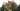The average SAT score of 920 breaks down into:

• SAT math: 470

The average ACT score for Finlandia University is 21 and their acceptance rate is 37.7%.

## Eastern Michigan University SAT scores

The average SAT score for Eastern Michigan University is 1050.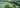The average SAT score of 1050 breaks down into:

• SAT math: 550

The average ACT score for Eastern Michigan University is 22 and their acceptance rate is 72.9%.

## Northern Michigan University SAT scores

The average SAT score for Northern Michigan University is 1050.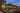The average SAT score of 1050 breaks down into:

• SAT math: 510

The average ACT score for Northern Michigan University is 23 and their acceptance rate is 74.4%.

## Ferris State University SAT scores

The average SAT score for Ferris State University is 1060.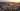The average SAT score of 1060 breaks down into:

• SAT math: 520

The average ACT score for Ferris State University is 22 and their acceptance rate is 74.2%.

## Western Michigan University SAT scores

The average SAT score for Western Michigan University is 1078.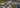The average SAT score of 1078 breaks down into:

• SAT math: 530

The average ACT score for Western Michigan University is 23 and their acceptance rate is 82.3%.

## University of Michigan-Flint SAT scores

The average SAT score for University of Michigan-Flint is 1081.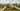The average SAT score of 1081 breaks down into:

• SAT math: 530

The average ACT score for University of Michigan-Flint is 23 and their acceptance rate is 65.1%.

## Cornerstone University SAT scores

The average SAT score for Cornerstone University is 1083.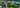The average SAT score of 1083 breaks down into:

• SAT math: 510

The average ACT score for Cornerstone University is 22 and their acceptance rate is 67.4%.

## Saginaw Valley State University SAT scores

The average SAT score for Saginaw Valley State University is 1092.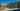The average SAT score of 1092 breaks down into:

• SAT math: 540

The average ACT score for Saginaw Valley State University is 23 and their acceptance rate is 77.4%.

## Central Michigan University SAT scores

The average SAT score for Central Michigan University is 1099.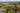The average SAT score of 1099 breaks down into:

• SAT math: 540

The average ACT score for Central Michigan University is 23 and their acceptance rate is 68.8%.

## College for Creative Studies SAT scores

The average SAT score for College for Creative Studies is 1110.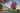The average SAT score of 1110 breaks down into:

• SAT math: 555